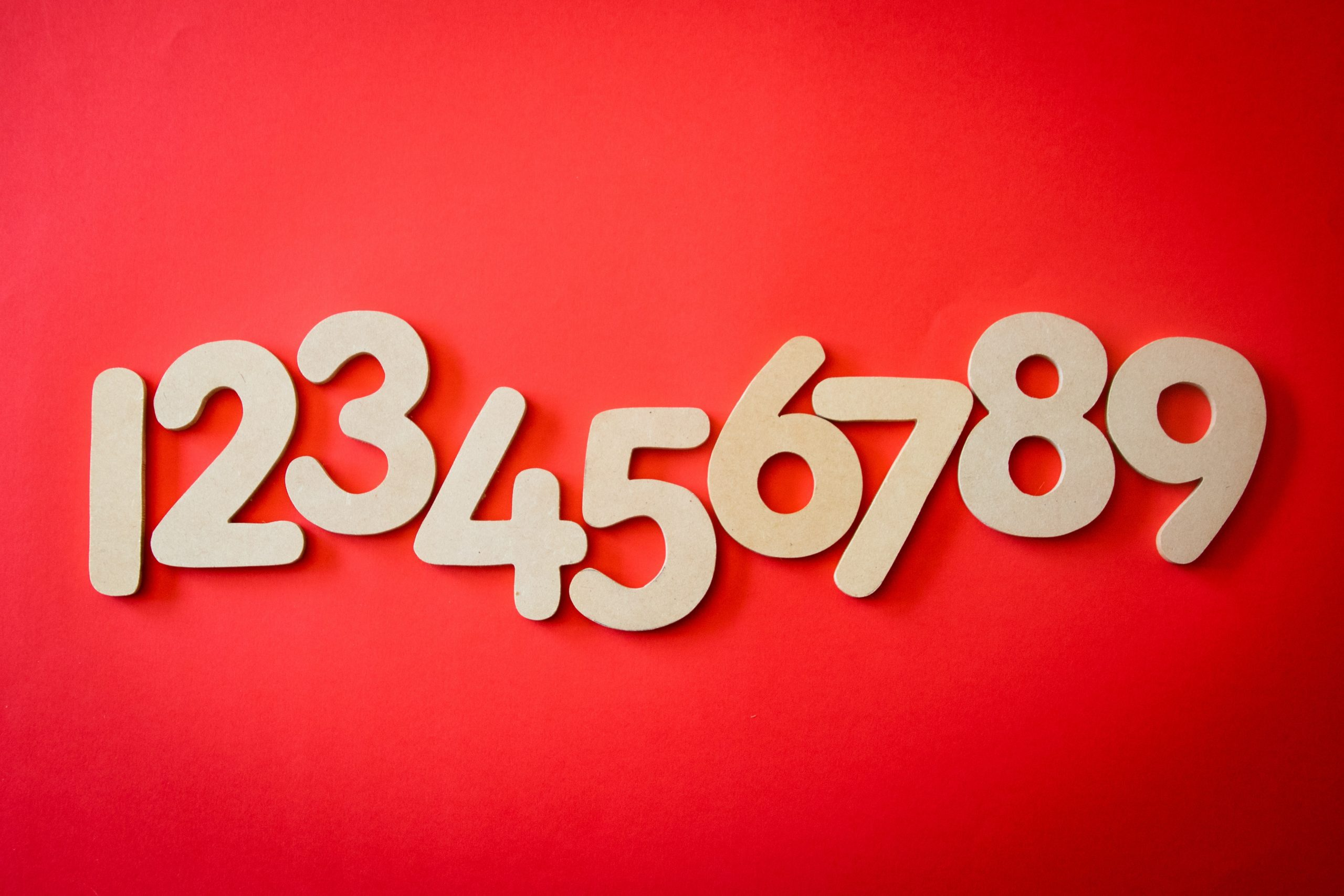# Learn To Use For Loops In C++In programming, one of the most used statement is for() loops. It is used to count in range with given conditions. If you know exactly how many times you want to execute a block of code in your loop, then they are very useful than other loops. Occasionally, it is used to calculate series in range or to list elements that have the number of elements (i.e. string lists, arrays, char arrays, vectors, structured arrays, etc. )

for() function is generally used with 3 parameters separated by ; character. the first one is initial-expression defines primary start condition (a=0 for example) and the second one is conditional expression defines looping condition ( for example a<10), this condition limits its range, and the third parameter is looping condition that defines the change in our loop statement in every loop.

We can create single-line for() loop as an example here,

We can define variable in the for() parameters as below.

or we can dive it to two lines,

these 3 examples above are same. If we have more than one line in our code block, then we must use { and } brackets, as here,

Note that in for loops last parameter has no ; character and never put ; at the end of for without statement like this for( i=0; i<10; i++ ); This example below will print out numbers between 0 and 10, including 0 and 10. The output will be 0,1,2,3,4,5,6,7,8,9,10

This code below will print out even numbers between 0 and 10, the output will be 0,2,4,6,8,10

Instead of a+2 term to increase we can use a = a+2 also as here, but remember this is slower than the previous one in operation,

This code below will print out odd numbers between 1 and 9, the output will be 1,3,5,7,9

and as same above we can also write like this too,

If you want to learn about more advanced usage and General Loop Statements in Modern C++ please check this post,## Oh hi there 👋It’s nice to meet you.

Reduce development time and get to market faster with RAD Studio, Delphi, or C++Builder.
Design. Code. Compile. Deploy.